# Regression with Cluster Effect¶

Oct. 18, 2018 $\newcommand{\bs}{\boldsymbol}$ $\newcommand{\argmin}{\underset{\bs{#1}}{\text{arg min}}\,}$ $\newcommand{\argmax}{\underset{\bs{#1}}{\text{arg max}}\,}$ $\newcommand{\tr}{^{\top}}$ $\newcommand{\norm}{\left|\left|\,#1\,\right|\right|}$ $\newcommand{\given}{\,|\,}$

Suppose that the population's underlying distribution has three clusters

$$y = \begin{cases} x + 16 + \varepsilon & \text{ if } x \text{ in cluster 1} \\ -x + 25 + \varepsilon & \text{ if } x \text{ in cluster 2}\\ -3x + 36 + \varepsilon & \text{ if } x \text{ in cluster 3}. \end{cases} \tag{1}$$

where $\epsilon$ follows a standard normal distribution. A priori, let's assume that we do not know this distribution, so we only use it to generate our data. We can think of the clusters as distinct geographical regions with distinct characteristics.

This is implemented by the following function.

In :
import numpy as np
import matplotlib.pyplot as plt

In :
def load_data(m=300, seed=1, clusters=3):
np.random.seed(seed)

N = int(m/clusters) # number of points per cluster

X = np.zeros((m, 2)) # data matrix where each row is a single example
Y = np.zeros(m) # labels vector (-1 for red, 1 for blue)

for j in range(clusters):
ix = range(N*j,N*(j+1)) # index by cluster
X1 = np.random.normal(4, 2, N)
X2 = np.full((N, 1), j)
X[ix] = np.c_[X1, X2]
Y[ix] = X1*(1-2*j) + (j+4)**2 + np.random.normal(0, 1, N)

return X, Y

In :
X, Y= load_data()
plt.scatter(X[:,0], Y, c=X[:,1], s=40, cmap=plt.cm.jet)
plt.show()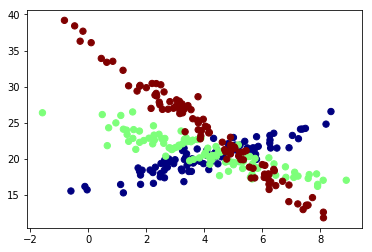For this model, since we know the underlying distribution, we know that the Bayes' optimal error should only come from the term $\varepsilon$ defined in (1).

## Simple Linear Regression¶

The most naive way is to fit a linear regression to the data without taking into consideration of the clustering.

In :
from sklearn.linear_model import LinearRegression

In :
lm1 = LinearRegression()
lm1.fit(X[:,0].reshape(-1, 1), Y)

print('Slope of the line is', lm1.coef_)
print('Intercept is', lm1.intercept_)
print('The R squared is', lm1.score(X[:,0].reshape(-1, 1), Y))

plt.scatter(X[:,0], Y, c=X[:,1], s=40, cmap=plt.cm.jet)
x = np.linspace(-2, 8, 100)
y = lm1.coef_*x + lm1.intercept_
plt.plot(x, y, c='red')
plt.show()

Slope of the line is -1.1731825980973616
Intercept is 26.45594285392711
The R squared is 0.28722584160908804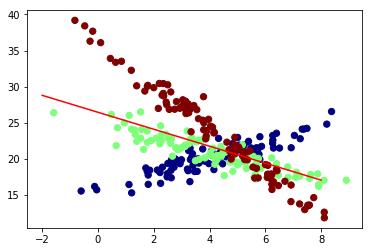The $R^2$ is not so good. This means that the simple linear regression only captures 28.7% of the total variance of the data.

## Multiple Linear Regression¶

The most natural alternative is to use a multiple linear regression, while encoding each cluster index using one-hot encoder. In our case, the index label $\{0, 1, 2\}$ will be encoded as vectors:

$$\begin{bmatrix} 1 \\ 0 \\ 0 \end{bmatrix}, \quad \begin{bmatrix} 0 \\ 1 \\ 0 \end{bmatrix}, \quad \begin{bmatrix} 0 \\ 0 \\ 1 \end{bmatrix}.$$

In :
from sklearn.preprocessing import OneHotEncoder

In :
enc = OneHotEncoder()

# Taking the first two columns as the third is redundant
onehot = enc.fit_transform(X[:,1].reshape(-1, 1)).toarray()

# Deleting the last column and adding the one-hot encoded columns
X_new = np.delete(X, 1, axis=1)
X_onehot = np.concatenate([X_new, onehot], axis=1)

In :
# Print 5 random rows of the matrix
np.random.seed(3)
index = np.random.randint(0, len(X_onehot)+1, 5)
print(X_onehot[index, :])

[[-1.58617     0.          1.          0.        ]
[ 6.91621648  0.          0.          1.        ]
[ 4.40366036  0.          1.          0.        ]
[ 2.35506562  0.          0.          1.        ]
[ 1.19534171  0.          0.          1.        ]]


Note that even though the third column is redundant, sklearn can deal with this effectively, so we do not need to remove this column. Including this column improves the interpretability of our model.

In :
lm = LinearRegression()
lm.fit(X_onehot, Y)
print('Coefficients are', lm.coef_)
print('Intercept is', lm.intercept_)
print('The R squared is', lm.score(X_onehot, Y))

Coefficients are [-1.18148935 -1.34684223 -0.78075603  2.12759825]
Intercept is 26.48991608156099
The R squared is 0.4136584295687384


We see that the model's performance improves slightly, but still less than desirable. This is surprising to me at first since the underlying distribution of the data is linear. However, when you think about it more, you realize that combining three linear clusters may not always produce a linear surface! Let's visualize this in 3D space.

In :
from mpl_toolkits.mplot3d import Axes3D

fig = plt.figure(figsize=[10, 10])

# Plotting the new data in 3D
ax.scatter(X[:,0], X[:,1], Y, c=X[:,1], s=40, cmap=plt.cm.jet)
ax.set_xlabel('X')
ax.set_ylabel('Cluster')
ax.set_zlabel('Y')
ax.view_init(10, 70)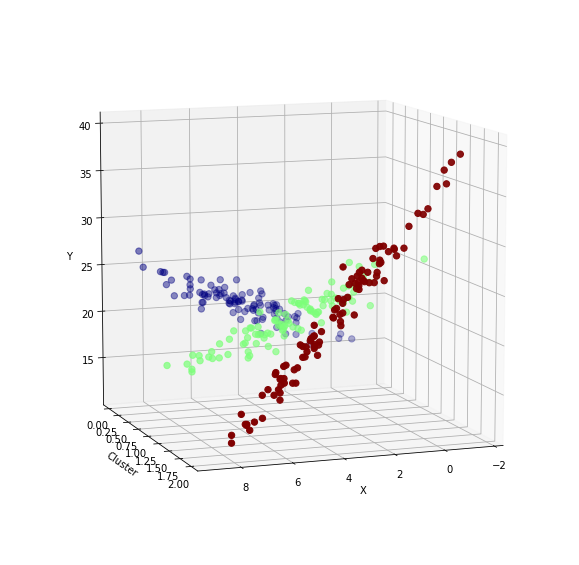Indeed there does not exist a "plane" (in 4D space) that can well interpolate the data!

## Support Vector Regression¶

The Support Vector Regression (SVR) is similar to Support Vector Classifier. The SVR solves the following convex optimization problem.

\begin{aligned} \min_{\bs{w}, b, \xi, \xi^*} & \frac{1}{2}\bs{w}\tr \bs{w} + C\sum_{i=1}^n (\xi_i + \xi_i^*) \\ \text{subject to: }\quad & y_i-\bs{w}\tr \phi(\bs{x}_i) - b \leq \varepsilon + \xi_i, \\ & \bs{w}\tr \phi(\bs{x}_i) +b-y_i \geq \varepsilon + \xi_i^*,\\ & \xi_i, \xi_i^* \geq 0, \,\, i=1,..., n. \end{aligned}

where $\phi(\bs{x})$ defines a feature map that defines the kernel

$$K(\bs{x}, \bs{y}) = \phi(\bs{x})\tr \phi(\bs{y})$$

The most popular kernel function is the Radial Basis Function (rbf):

$$K(\bs{x}, \bs{y}) = \text{exp}\left( - \gamma \norm{\bs{x}-\bs{y}}^2\right).$$

The nonnegative constant $\gamma$ is a parameter we can tune, just like $C$.

Since this post is a quick survey of different regression techniques, we will not dive into the rich theory behind this method. We can simply use sklearn to quickly implement this.

In :
from sklearn.svm import SVR

clf = SVR()
clf.fit(X_onehot, Y)
print('The R squared is', clf.score(X_onehot, Y))

plt.scatter(X[:,0], Y, c=X[:,1], s=40, cmap=plt.cm.jet)
plt.scatter(X[:,0], clf.predict(X_onehot))
plt.show()

The R squared is 0.8135847633424437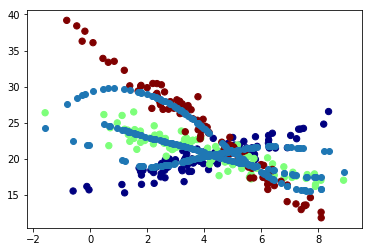Using the default version of SVR, we are already doing much much better than previous methods. To make it even better, let's do some parameter tuning by writing a GridSearch function as follows.

In :
import pandas as pd
import seaborn as sns
import itertools

def GridSearch(parameters):
"""
parameters: must be a dictionary of the form
{"C: [], "gamma": []}
"""
scores, C, gamma = [], [], []

for param in itertools.product(*parameters.values()):
clf = SVR(C=param, gamma=param)
clf.fit(X_onehot, Y)
scores.append(clf.score(X_onehot, Y))
C.append(param)
gamma.append(param)

df = pd.DataFrame({'C': C, 'gamma': gamma, 'score': scores})
best_index = scores.index(max(scores))

return C[best_index], gamma[best_index], scores[best_index]

In :
param_grid = {'C': [0.01, 0.1, 10], 'gamma': [1, 0.1, 0.01, 0.001, 0.0001]}
GridSearch(param_grid)

Out:
(10, 1, 0.947501887509181)
In :
clf = SVR(C=10, gamma=1)
clf.fit(X_onehot, Y)
print('The R squared is', clf.score(X_onehot, Y))

plt.scatter(X[:,0], Y, c=X[:,1], s=40, cmap=plt.cm.jet)
plt.scatter(X[:,0], clf.predict(X_onehot))
plt.show()

The R squared is 0.947501887509181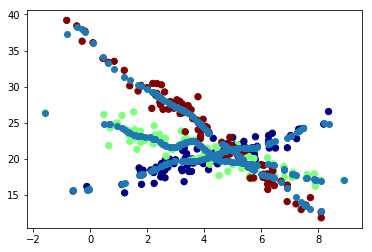Finally, we must beware that the SVR has more than enough flexibility to overfit the data by simply using a much larger value of $C$ and $\gamma$.

In :
clf = SVR(C=1000, gamma=1000)
clf.fit(X_onehot, Y)
print('The R squared is', clf.score(X_onehot, Y))

plt.scatter(X[:,0], Y, c=X[:,1], s=40, cmap=plt.cm.jet)
plt.scatter(X[:,0], clf.predict(X_onehot))
plt.show()

The R squared is 0.9965061983708676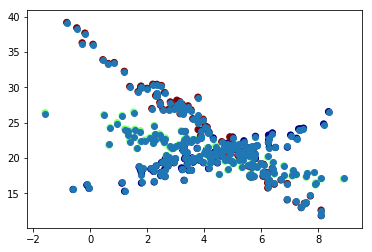This is a classic example of overfitting. When the error is smaller thant the Bayes' error, the model is picking up unnecessary random effects.